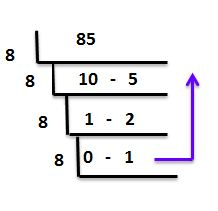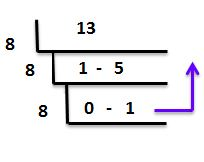# Binary to Octal Conversion

In binary to octal conversion, we learn to convert base 2 number system into base 8 number system. We cannot directly convert binary to octal, so we first convert binary to decimal, then the decimal number to the equivalent octal number system. Binary numbers are commonly used in computers, in the form of bits and bytes, since the computer understand the language of 0 and 1 only. At the same time, octal numbers are used in electronics. Before going to the conversion, we have to learn about octal and binary numbers.

What are Binary Numbers?

Numbers to base 2 is called binary numbers. It uses only two digits, 0 and 1. It is denoted by a2, where a is a number with 0’s and 1’s.

Examples:

• 1111102
• 11111112
• 10110012

What are Octal numbers?

The number to the base 8 is called octal numbers. It uses the numbers from 0 to 7. The numbers 8 and 9 are not included in the octal number system. It is denoted by a8 where a is a number with digits 0 to 7.

Examples:

• 21458
• 71658
• 468

## Conversion from Binary to Octal

In number system, you will come across different types of numbers such as binary, octal, decimal and hexadecimal. To convert binary numbers to octal numbers, follow the below steps:

• Take the given binary number
• Multiply each digit by 2n-1 where n is the position of the digit from the decimal
• The resultant is the equivalent decimal number for the given binary number
• Divide the decimal number by 8
• Note the remainder
• Continue the above two steps with the quotient till the quotient is zero
• Write the remainder in the reverse order
• The resultant is the required octal number for the given binary number

Here is a table for decimal number and equivalent octal number, to solve the problems based on their conversion more quickly.

 Decimal Number Octal Number 0 0 1 01 2 010 3 011 4 100 5 101 6 110 7 111

### Binary to Octal conversion Examples

Example 1: Convert 10101012 to octal

Solution:

Given binary number is 10101012

First, we convert given binary to decimal

10101012 = (1 * 26) + (0 * 25 ) + (1 * 24) + (0 * 23) + (1 * 22) + (0 * 21) + (1 * 20)

= 64 + 0 + 16 + 0 + 4 + 0 + 1

= 64 + 21

0101012= 85 (Decimal form)

Now we will convert this decimal to octal formTherefore, the equivalent octal number is 1258.

Example 2: Convert 011012 to octal

Solution:

Given binary number is 011012

First we convert given binary to decimal

011012 = (0 * 24) + (1 * 23) + (1 * 23) + (0 * 2) + (1 *20)

= 0 + 8 + 4 + 0 +1

011012= 13 (Decimal form)

Now we will convert this decimal to octal formTherefore, the equivalent octal number is 158.

Register with BYJU’S – The Learning App for more Maths-related concepts and explore exciting videos.

Quiz on Binary to Octal